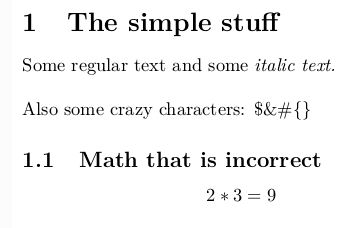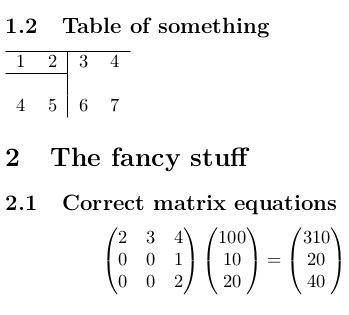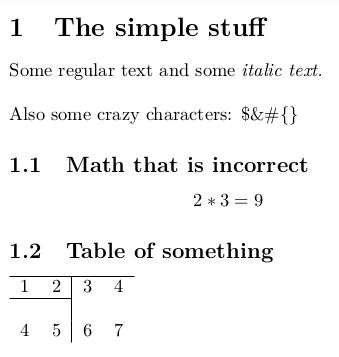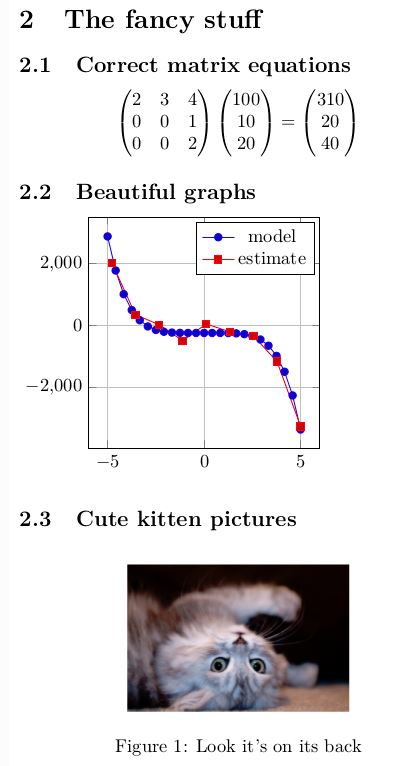# Pylatex module in python

• Difficulty Level : Basic
• Last Updated : 11 Jan, 2019

Latex :

Latex pronounced as “Lay-tech” is a document making system for high-quality documentation. It is mostly used for technical or scientific document preparation but it can be used for almost all forms of publishing. Latex is not a word processor like MS Word or LibreOffice Writer. Instead, Latex encourages authors not to worry about the look of their documents but to concentrate on getting the right content. For example, consider the below document:

This article explains the use of pylatex module
GeeksforGeeks
October 2018


To produce this in most word processors, the author would have to decide what layout to use, so would select (suppose) 18pt Helvetica for the title, 12pt Times Roman for the name, and so on. This results into author wasting their time designing the document. Latex is based on the idea that let authors get on with writing the document and leave the designing of the document to document designers. So, in Latex, you would input the above document as:

\documentclass{article}
\title{This article explains use of pylatex module}
\author{GeeksforGeeks}
\date{October 2018}
\begin{document}
\maketitle
\end{document}


Layout of a latex document :
There are two main parts of a latex document:
Preamble :

• Preamble is the first part of a latex file.
• It contains details about the document such as Document class, author name, title etc

Body :

• In the body part of a latex document, sections, tables, mathematical equations, graphs etc can be included
• All the contents of the document are within a ‘\begin{document}’ and a ‘\end{document}’

Some features of Latex are:

1. Preparing journal articles, technical reports, technical or non-technical books, and also slide presentations.
2. It provides better control over large documents containing sectioning, references, tables and figures.
3. It can also be useful for preparing documents containing complex mathematical formulas.
4. Generation of bibliographies and indexes is automatic in LaTeX.
5. It also provides multi-lingual typesetting support.
6. In a latex document we can also add graphics, artwork, and process or spot colour.
7. Usage of PostScript or metafont fonts is also possible in LaTeX.

Example of a LaTeX document:
Example 1: In this example we form a simple latex in order to from latex, we used simple input format as we used in latex.

 \documentclass{article}%\usepackage[T1]{fontenc}%\usepackage[utf8]{inputenc}%\usepackage{lmodern}%\usepackage{textcomp}%\usepackage{lastpage}%\usepackage[tmargin=1cm, lmargin=10cm]{geometry}%\usepackage{amsmath}%\usepackage{tikz}%\usepackage{pgfplots}%\pgfplotsset{compat=newest}%\usepackage{graphicx}%%%%\begin{document}%\normalsize%\section{The regular stuff}%\label{sec:The regular stuff}%Some text and some%\textit{italic text. }%\newline%Also some crazy symbols: \$\&\#\{\}%\subsection{Incorrect math}%\label{subsec:Incorrect math}%\[%2*3 = 22%\]  %\end{document} Output:Example 2: In this example we used, label, subsection in order to form a latex.  \documentclass{article}%\usepackage[T1]{fontenc}%\usepackage[utf8]{inputenc}%\usepackage{lmodern}%\usepackage{textcomp}%\usepackage{lastpage}%\usepackage[tmargin=1cm, lmargin=10cm]{geometry}%\usepackage{amsmath}%\usepackage{tikz}%\usepackage{pgfplots}%\pgfplotsset{compat=newest}%\usepackage{graphicx}%%%%%\subsection{Table}%\label{subsec:Table}%\begin{tabular}{rc|cl}%\hline%a&b&c&d\\%\cline{1%-%2}%&&&\\%e&f&g&7h\\%\end{tabular}  %\section{Special features}%\label{sec:Special features}%\subsection{Correct matrix equations}%\label{subsec:Correct matrix equations}%\[%\begin{pmatrix}%1&4&4\\%2&3&4\\%2&2&5%\end{pmatrix} \begin{pmatrix}%800\\%30\\%30%\end{pmatrix} = \begin{pmatrix}%810\\%60\\%50%\end{pmatrix}%\]  %\end{document} Output :What is Pylatex : PyLaTeX is a Python library for creating and compiling latex documents. The goal of this library is to be easy but is also to provide an extensible interface between Python and latex. Some features of pylatex are: • We can access all the features of LaTeX in python using this module • We can make documents with fewer lines of code • Since python is a high-level language it is easier to write code for pylatex in python as compared to LaTeX • In the above LaTeX code you must have seen that to give equations we have to calculate values and then input in LaTeX document but with python’s added functionality of performing arithmetic operations it is much easier to prepare documents Create a Pylatex document : • Install MikTeX and pylatex module in your system and import it into python code. For installing MikTeX on your system, go to : https://miktex.org/download  For installing pylatex on windows based operating system, enter the following command in command prompt: python -m pip install pylatex  • To create a document import document class from pylatex module. In latex there are different document types : article, report, letter etc. To create a document of the type article, create an object of the Document class of latex and as an argument pass ‘article’ doc=Document(documentclass='article')  • To add the necessary changes in the document such as styling or formatting, import the classes required in the python code from pylatex. To add different utilities in a latex document using pylatex the following way is feasible from pylatex import Document, Section, Subsection from pylatex.utils import italic, bold  • To generate PDF file of the document, call the generate_pdf method of the Document class using the object of Document class and make sure to pass the name of the pdf document in its argument in this way doc.generate_pdf("Demo_article")  Pylatex Example : Code 1:  # Python program creating a# small document using pylatex  import numpy as np  # importing from a pylatex modulefrom pylatex import Document, Section, Subsection, Tabularfrom pylatex import Math, TikZ, Axis, Plot, Figure, Matrix, Alignatfrom pylatex.utils import italicimport os  if __name__ == '__main__': image_filename = os.path.join(os.path.dirname(__file__), 'kitten.jpg')   geometry_options = {"tmargin": "1cm", "lmargin": "10cm"} doc = Document(geometry_options=geometry_options)   # creating a pdf with title "the simple stuff" with doc.create(Section('The simple stuff')): doc.append('Some regular text and some') doc.append(italic('italic text. ')) doc.append('\nAlso some crazy characters:$&#{}')        with doc.create(Subsection('Math that is incorrect')):            doc.append(Math(data=['2*3', '=', 9]))          # creating subsection of a pdf        with doc.create(Subsection('Table of something')):            with doc.create(Tabular('rc|cl')) as table:                table.add_hline()                table.add_row((1, 2, 3, 4))                table.add_hline(1, 2)                table.add_empty_row()                table.add_row((4, 5, 6, 7))       # making a pdf using .generate_pdf    doc.generate_pdf('full', clean_tex=False)

Output:Code 2:

 import numpy as np  from pylatex import Document, Section, Subsection, Tabularfrom pylatex import Math, TikZ, Axis, Plot, Figure, Matrix, Alignatfrom pylatex.utils import italicimport os  if __name__ == '__main__':    image_filename = os.path.join(os.path.dirname(__file__), 'kitten.jpg')      geometry_options = {"tmargin": "1cm", "lmargin": "10cm"}    doc = Document(geometry_options=geometry_options)      # making a matrix using numpy module    a = np.array([[100, 10, 20]]).T    M = np.matrix([[2, 3, 4],                   [0, 0, 1],                   [0, 0, 2]])      # creating a title using "the fancy stuff"    with doc.create(Section('The fancy stuff')):        with doc.create(Subsection('Correct matrix equations')):            doc.append(Math(data=[Matrix(M), Matrix(a), '=', Matrix(M * a)]))          # creating a subsection of pdf        with doc.create(Subsection('Alignat math environment')):            with doc.create(Alignat(numbering=False, escape=False)) as agn:                agn.append(r'\frac{a}{b} &= 0 \\')                agn.extend([Matrix(M), Matrix(a), '&=', Matrix(M * a)])          with doc.create(Subsection('Beautiful graphs')):            with doc.create(TikZ()):                plot_options = 'height=4cm, width=6cm, grid=major'                with doc.create(Axis(options=plot_options)) as plot:                    plot.append(Plot(name='model', func='-x^5 - 242'))                      coordinates = [                        (-4.77778, 2027.60977),                        (-3.55556, 347.84069),                        (-2.33333, 22.58953),                        (-1.11111, -493.50066),                        (0.11111, 46.66082),                        (1.33333, -205.56286),                        (2.55556, -341.40638),                        (3.77778, -1169.24780),                        (5.00000, -3269.56775),                    ]                      plot.append(Plot(name='estimate', coordinates=coordinates))          with doc.create(Subsection('Cute kitten pictures')):            with doc.create(Figure(position='h!')) as kitten_pic:                kitten_pic.add_image(image_filename, width='120px')                kitten_pic.add_caption('Look it\'s on its back')      # Creating a pdf    doc.generate_pdf('full', clean_tex=False)

Output :My Personal Notes arrow_drop_up